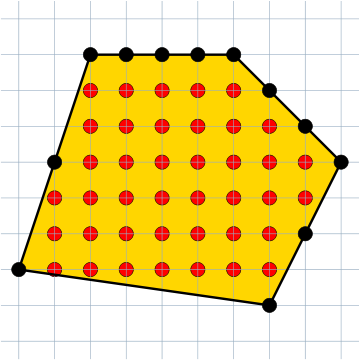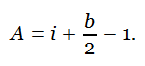# Pick’s TheoremGeorg Alexander Pick found a useful way to determine the area of a simple polygon with integer coordinates. If i is the number of lattice points in the interior and b is the number of lattice points on the boundary, then the area is given byThere are 40 lattice points in the interior of the figure above and 12 on the boundary, so its area is 40 + 12/2 – 1 = 45.

(Thanks, Pål.)